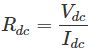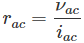# Diode Resistance

Resistance is the opposition offered to the flow of current through the device. Hence, diode resistance can be defined as the effective opposition offered by the diode to the flow of current through it. Ideally speaking, a diode is expected to offer zero resistance when forward biased and infinite resistance when reverse biased. However, no device can ever be ideal. Thus, practically speaking, every diode is seen to offer a small resistance when forward biased, and a considerable resistance when reverse biased. One can characterise the given diode regarding its forward and reverse resistances.

## Forward Resistance

Even after forward biasing, the diode will not conduct until it reaches a minimum threshold voltage level. After the applied voltage exceeds this threshold level, the diode starts to conduct. We refer the resistance, offered by the diode under this condition as the forward resistance of the diode. That is, the forward resistance is nothing but the resistance offered by the diode when the diode is working in its forward biased condition.

Forward resistance is classified into two types viz., static or dynamic depending on whether the current flowing through the device is DC (Direct Current) or AC (Alternating Current), respectively.### Static or DC Resistance

It is the resistance offered by the diode to the flow of DC through it when we apply a DC voltage to it. Mathematically the static resistance is expressed as the ratio of DC voltage applied across the diode terminals to the DC flowing through it (shown by the black dotted line in Figure 1) i.e.### Dynamic or AC Resistance

It is the resistance offered by the diode to the flow of AC through it when we connect it in a circuit which has an AC voltage source as an active circuit element. Mathematically the dynamic resistance is given as the ratio of change in voltage applied across the diode to the resulting change in the current flowing through it. This is shown by the slope-indicating red solid lines in Figure 1 and is expressed as## Reverse Resistance

When we connect the diode in reverse biased condition, there will be a small current flowing through it which is called the reverse leakage current. We can attribute the cause behind this to the fact that when the diode functions in its reverse mode, it will not be completely free of charge carriers. That is, even in this state, one can experience the flow of minority carriers through the device.
Due to this current flow, the diode exhibits reverse resistance characteristic which is shown by the purple dotted line in Figure 1. The mathematical expression for the same is similar to that for the forward resistance and is given byWhere, Vr and Ir are the reverse voltage and the reverse current respectively.
After knowing the basic facts about the diode resistance, it is important to note the fact that
“Generally the diodes have a high ratio of reverse to forward resistance, which makes them essentially unidirectional in function”.

Want To Learn Faster? 🎓
Get electrical articles delivered to your inbox every week.
No credit card required—it’s 100% free.